# Pope Benedict XVI - Mark 666

(Copyright © April 4th, 2005 -    All rights reserved)

Paradoxically, at the proper moment of his agony, John Paul II or better John Paul Le Grand, claims " I am happy ". He is certainly right because he had the genius to bet on the lively forces of tomorrow, that is on the young people to whom he reserved his all last words.

In the young people, he planted his tree from which the best fruits are to come, making the Pope's work ceaselessly renewable. What a big visionary! It is important to underline that mysteriously the life and the death of John Paul II are both strongly marked by the number 13!

First, the date of its death corresponds exactly to the numerical code 13:
Death on April 2nd, 2005 or 04/02/2005         4 + 2 + 2 + 5 = 13

Even the precise hour of its death wears the mark 13:
Death arisen at 9:37 pm or , 21h 37 , the Vatican time         2 + 1 + 3 + 7 = 13
• Year week at its death : 13th week of year 2005 (Death arisen at the start of April)
• Age at its death 85 years (1920-2005)         8 + 5 = 13
• His successor Benedict XVI (Cardinal Joseph Ratzinger) is the 265th Pontiff of the Roman Catholic Church
265         2 + 6 + 5 = 13
• Week day of the appearances at Fatima of his idol Mary: always the 13th of the month
• Karol Józef Wojtyla (Pope John Paul II) was born on May 18, 1920 :
• 05/18/1920         5 + 1 + 8 + 1 + 9 + 2 = 26 = 13 x 2
• In the numerology Starter 13, that is A=13, B=14, C=15, ... , Y=37, Z=38, we have:
Karol Józef Wojtyla = 429 = 13 x 33
John Paul II = 193 + 2 = 195 = 13 x 15
• His mother died on April 13th, 1929 .
• October 13, 1946 Karol Wojtyla became Sub-diaconate
• On January 13, 1964, he was nominated Archbishop of Cracow by Pope Paul VI, who made him a cardinal June 26 [13 x 2], 1967 .
• Elected Pope on October 16, 1978 , at the age of 58 years         5 + 8 = 13
• John Paul II was first non-Roman Pope since 455 years         455 = 35 x 13
• The attempt against the Pope Jean-Paul II took place on May 13th, 1981 , at the place Saint Pierre.
• The young turk Mehmet Alì Agca who made an attempt on the Pope's life has 13 letters in his full name.
• His pontificate lasted 26 years, that is 13 x 2.
• He leaves behind him 117 cardinals-voters who will have to choose his successor by two-thirds majority; 117 = 9 x 13
• On April 4th, 2005, was held the very first meeting of the cardinals after the death of the Pope; the number of cardinals present in this important session was 65, that is 5 x 13.
• Cardinals have fixed to April 18 the starting date of the Conclave for election of the new pope, at the Sistine Chapel (13 letters); April 18 or 04/18         4 + 1 + 8 = 13
• The election of the new Pope Benedict XVI (born Jozef Ratzinger on April 16, 1927) was announced on April 19, 2005 by:
*   White smoke began to pour from the Sistine Chapel's chimney at 5:50 p.m. (Vatican time); but at that time no bells are heard confirming a pope's election, contrary to the planned simultaneous occurence.
5:50 p.m. or 17h 50         1 + 7 + 5 + 0 = 13
*   At 6:04 p.m., about 15 minutes after the smoke began to rise out, the bells of the basilica began to peal.
6:04 p.m. or 18h 04         1 + 8 + 0 + 4 = 13
• Benedict XVI, elected at age 78 , is the oldest cardinal to become pope since Clement XII in 1730, who, like Ratzinger, was elected at age 78.
78 = 13 x 6
At the time of the 2005 Conclave, Ratzinger was one of only 14 remaining cardinals appointed by Paul VI, and one of only three of those under the age of 80 and thus eligible to participate in that conclave.
• In the basic ordinal numerology (A=1, B=2, C=3, ... , Y=25, Z=26):
Benedict = 62 and Benedict XVI = 62 + 16 = 78 = 13 x 6
French Benoît = 65 = 13 x 5
• Besides, in Starter 13 alphanumeric encoding (A=13, B=14, C=15, ... , Y=37, Z=38):
Josephum Ratzinger = 429 = 13 x 33   (Josephum is Latin for Joseph)
• Ratzinger was appointed Archbishop of Munich on May 28, 1977, and he was subsequently made a Cardinal by Pope Paul VI on June 27, 1977.
*   May 28, 1977 or 05/28/1977         5 + 2 + 8 + 1 + 9 + 7 + 7 = 39 = 13 x 3
*   June 27, 1977 or 06/27/1977         6 + 2 + 7 + 1 + 9 + 7 + 7 = 39 = 13 x 3
• His Holiness Pope Benedict XVI is the first German pontiff since 949 years; the last German pope being Victor II (born Gebhard, Count of Calw, pope from 1055 to 1057).
949 = 13 x 73
• Before his first appearance at the balcony of Saint Peter's Basilica after becoming pope, he was announced by the Cardinal Medina Estévez, protodeacon of the College of Cardinals.
Medina Estévez = 13 letters
• On Friday 13th May 2005, spoking in Latin at the basilica of St John Lateran, Pope Benedict XVI announced that the rule of waiting five years after a potential saint's death before the initial process of beatification can start was to be waived in the case of his predecessor John Paul II [see Benedict XVI paves way for John Paul to be made a saint, by Peter Popham in The Independent News Paper and Vatican, Pope'speech to the Clergy of Rome (May 13, 2005), DISCORSO DI SUA SANTITÀ BENEDETTO XVI AL CLERO DI ROMA ]. Pope Benedict's decision to accelerate the process for beatifying Karol Wojtyla came on the 24th anniversary of the attempted assassination of John Paul II by Mehmet Alì Agca, which also happens to be the saint's day of the Madonna of Fatima, whose devotion was close to the late pontiff's heart.

Panzer-kardinal, Benedictum XVI and 666
Preface and Warning: Rehabilitation of 666

Golden Number    Φ = 2sin 666º
666 = 73 π (Φ -1) = 73 π φ     or     666 = (6/5) 73 Φ
with     π = 3.141593     Φ = 1.618034     φ = Φ -1 = 0.618034

Curiously, I have found recently a direct link between the Beast Number 666 and the famous Golden Number Φ, well-known by Pythagoras, Leonardo da Vinci ... and in Sacred Geometry. This unexpected and incredible relation 666 versus Φ may constitute a way for the Rehabilitation of 666 !!! ...

Definition of the "Divine proportion" or Golden Ratio

The 3 irrational, transcendental numbers π , e and Φ are very important in Sacred Geometry. By definition, the Golden Number is the solution of the quadratic equation Φ2 + Φ -1 or the ratio of sequential entries of Fibonacci Numbers Fn The solution of the former equation is (-1 ± √5)/2 so that:
Φ = 1.61803 39887 49894 84820 45868 34365 63811 77203 09180...
φ = Φ -1 = 0.61803 39887 49894 84820 45868 34365 63811 77203 09180...
Φ + φ = √5
This relationship between Φ, φ and √5 can refer to David's star (5-pointed star) which form an inscribed five-sized regular pentagon.

Main propreties of irrational numbers π , e and Φ

Φ = 1/Φ + 1 = φ + 1 = 1/φ
1/Φ = φ = Φ - 1
Φ x φ = 1
Φ/φ = Φ + 1     and     φ/Φ = 1 - φ
Φ = φ + 1 = √5 - φ     and     φ = Φ - 1 = √5 - Φ
Φ2 = 1 + Φ     and     φ2 = 1 - φ
π ≈ 4/√Φ     and     π ≈ (6/5)Φ2
From Dixon (1991): 6/5(1 + Φ) = 3.141640... = π
45º = π/4 ≈ √Φ ≈ 1 - 1/3 + 1/5 - 1/7 + 1/9 ... = 0,7853981634 ...
π = 3.14159 26535 89793 23846 26433 83279 50288 41971 69399 37510 58209 74944 59230 ...
e = ∑1/n! = 2 + 1/2! + 1/3! + 1/4! + 1/5! + 1/6! + 1/7! + 1/8! + 1/9! + ...
by definition factorial n or n! = 1x2x3x ... x(n-1)xn
e = 2.71828 18284 59045 23536 02874 71352 66249 77572 47093 69996 ...
e ≈ 10 (√Φ -1)
Gelfond's constant eπ = (e)-1 = ( -1)-i
eπ = 23.140692632     and     eπ - π = 19.999099979... ≈ 20 ≈ Whole number
eπ ≈ πe     and     eπ ≈ π + 20     also     π2 ≈ 10     and     π3 ≈ 31
By interpreting the decimal point in π like a sign of multiplication and by considering per pairs the first ten decimals of π, Eli Eshoh clarifies the link with number 666:
π = 3.1415926536 ...         3x(14+15+92+65+36) = 3 x 222 = 666

Fibonacci numbers

Definition of Fibonacci numbers: Fn = Fn-1 + Fn-2     with     F0 = 0, F1 = 1, F2 = 1 ,
Fibonacci series:
0,1,1,2,3,5,8,13,21,34,55,89,144,233,377,610,987,1597,2584,4181,6765,10946,17711,28657, 46368,75025,121393,196418,317811,514229,832040,1346269,2178309,3524578,5702887, ...
The standard formula for the Fibonacci numbers is due to a French mathematician named Binet (1786-1856), who prove that the ratios of successive Fibonacci numbers, Fn+1/Fn, converges to Φ. The Binet Formula (1843) for Fn:
Fn+1/Fn = Φ = (1 + √5)/2
or    Fn = [Φn - (-φ)n]/√5     and    x = Fn+1/Fn = 1 + Fn/Fn+1 = 1 + 1/x
Guy (1990) notes some equivalence between Fibonacci and exponential series:
Fn = e(n-1)/2
e(n-1)/2     gives Fibonacci series 1,1,2,3,5,8,13,21,34,55,89,144,233,377,610,987,1597,2584 ...
for n = 0, 1, 2, 3, ...
1 mile ≈ Φ kilometers
Fn mi = Fn+1 km         5 mi = 8 km    or    8 mi = 13 km

Direct link between Beast Number 666 and Golden Number Φ

### Special Numbers 36, 144, 216 and 324

All are multiple of 18 :
36 = 2 x 18  144 = 8 x 18  216 = 12 x 18  324 = 18 x 18  666 = 37 x 18
Circle = 360º = 2 π radians         = 2 π/360 radians = π/180 radians     then:
36º = π/5     144º = 4π/5     216º = 6π/5     324º = 9π/5     666º = 37π/10
36 = 6 x 6 = 13 + 23 + 33 = 12 x 22 x 32 = (1 + 2 + 3)2
The sum of the first 36 numbers is equal to 666:

 1+2+3+4+5+6+7+8+9+10+11+12+13+14+15+16+17+18 +19+20+21+22+23+24+25+26+27+28+29+30+31+32+33+34+35+36 = 666

36=3!x6  (Triple 6...)   36=13+23+33  (Trinity...)  36=22x(1!+2!+3!)
"Mirror" of 36 = 1/36 = 0.27 777 777 777 ...         777
(1+6+7+9+6+1+6)4 = 364 = 1 679 616
144 = 36 x 4     216 = 36 x 6     324 = 36 x 9
144 = [(6+6) x (6+6)] = 3! x 4! = (1 x 2 x 3) x (1 x 2 x 3 x 4) = 6x6x4 = 6x24
144 = 12 x 12 = 122 and 441 = 21 x 21 = 212
441 = mirror of 144 and 21 = mirror of 12 ... like in 12 apostles;
21 and 12         2+1 = 1+2 = 3 ... like in Trinity
God created the world in 6 days, that is 6 x 24 hours = 144 hours

216 = 63 = 6x6x6     216 x 666.66666666666... = 144,000
144,000 ÷ 666 = 216.216 216 216 216 216 216...
According to the Bible, only 144,000 people from Israel, all with God's seal on their forehead, will be saved (Revelation 7:4)
666 is the sum of the first 144 digits of the decimal expansion of π
The beast number is equal to the sum of the squares of the first 7 primes
666 = 22 + 32 + 52 + 72 + 112 + 132 + 172
666 = 16 - 26 + 36

### Formulas for Link 666 versus Φ

After computing some sines and cosines [a good on line Trigonometry calculator available here], expressed in Degrees and absolute values, we obtain:
Φ/2 = sin 666º = cos 324º = cos 216º = cos 144º = cos 36º = 0.80901699...
Φ = 2sin 666º = 2cos 324º = 2cos 216º = 2cos 144º = 2cos 36º = 1,61803399...
1/Φ = 2 sin 18º = φ = 0.61803398...    with    sin 18º = 0.309016994...
Φ = sin 666º + cos 216º = sin 666º + cos (6x6x6)º
Φ = cos 144º + cos 36º = cos [(6+6) x (6+6)]º + cos (6 x 6)º
Φ = sin 666º + cos 144º = sin 666º + cos [(6+6) x (6+6)]º
666 = 73 π (Φ -1) = 73 π φ     or     666 = (6/5) 73 Φ
with   36 = 2 x 18  144 = 8 x 18  216 = 12 x 18  324 = 18 x 18  666 = 37 x 18

Some useful references

666 Myth at   http://www.666myth.co.nr/
666 Mythe at   http://www.666mythe.co.nr/
Trigonometry calculator On line, by 1728 Software Systems, at   http://www.1728.com/trigcalc.htm
Dixon, R. "The Story of Pi (π)" §4.3 in Mathographics. New York: Dover, pp. 44-49 and 98-101, 1991.
Keith, M. "The Number 666" J. Recr. Math. 15, 85-87, 1982-1983.
From MathWorld, Golden Ratio at   http://mathworld.wolfram.com/GoldenRatio.html
Guy, R. K. "The Second Strong Law of Small Numbers" Math. Mag. 63, 3-20, 1990.
Fibonacci Numbers & The Golden Ratio Link Web Page
at   http://www.goldenratio.org/info/index.html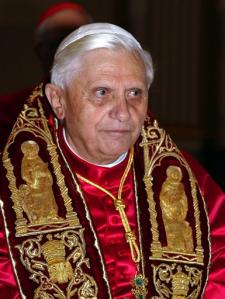Source: Associated Press / Osservatore Romano
Pope Benedict XVI prepares to leave the Sistine Chapel on his way to greet and bless the faithful
Cardinal Ratzinger was already one of the most influential men in the Vatican and a close associate of the late Pope John Paul II before he became Pope. He was the highest-ranking official in the Catholic Church, after John Paul II. He also presided over the funeral of John Paul II and the Conclave of April 2005 which elected him.

Cardinal Ratzinger was conservative and usually took inflexible and controversial views on topics such as homosexuality, gay marriage, birth control, abortion, priest's wedding and even the Turkey'entry in the European Community. So, his nickname became the Panzer-kardinal. Panzer is an abbreviation of the German designation for tanks or Panzerkampfwagen, using during World War II.

Actually, it is amazing to notice that Panzer-kardinal Ratzinger, now Pope Benedictum XVI, carry the mark of the beast 666 in many numerologies.
• The Panzer-kardinal = 666 in Telephone x9 system, a numerology multiple 9 of the basic alphanumeric encoding of your phone keyboard (A, B, C)=18=9x2; (D, E, F)=27=9x3; (G, H, I)=36=9x4; (J, K, L)=45=9x5; (M, N, O)=54=9x6; (P, R, S)=63=9x7; (T, U, V)=72=9x8; (W, X, Y)=81=9x9; Q=Z=0
(for details, see 666 Calculators at   http://www.666myth.co.nr/ )
• Panzer-kardinal = 1221, with 1221 x (6/11) = 666 in the Standard numerology: A=1, B=2, C=3, D=4, E=5, F=6, G=7, H=8, I=9, J=10, K=20, L=30, M=40, N=50, O=60, P=70, Q=80, R=90, S=100, T=200, U=300, V=400, W=500, X=600, Y=700, Z=800.
• Panzer-kardinal Jozef Ratzinger = 666 in Starter 13 encoding, where A=13, B=14, C=15, ... , Y=37, Z=38.
• Panzer-cardinal Joseph Ratzinger = 666 in Base 2 numerology (A=2, B=4=2x2, C=6=2x3, ... , Y=50=2x25, Z=52=2x26)
• Papa Benedictum XVI = 650 + 16 = 666 (Papa Benedictum XVI = 650) in Base 5 encoding with A=5, B=10=5x2, C=15=5x3, ... , Y=125=5x25, Z=130=5x26.
• In French, Benoît XVI = Benoît 16 = 650 + 16 = 666 in Base 10 numerology where A=10, B=20=10x2, C=30=10x3, ... , Y=250=10x25, Z=260=10x26.
• Pope Benedict = 666 in Starter 47 encoding: A=47, B=48, C=49, ... , Y=71, Z=72.
• Benedict the Sixteenth = 1776 in Suite A0 numerology, with 1776 x (3/8) = 666
In Suite A1 or s1 encoding, the values of letters of the alphabet are sums of series s1: A = 1, B=(1+2) = 3, C=(1+2+3) = 6, D=(1+2+3+4) = 10, ..., Z=(1+2+3+4+ ... +25+26) = 351. So, the numerology Suite A0 ou s0 is defined with link to A1 by s0 = s1 - 1; that means A = 0, B=(0+2) = 2, C=(0+2+3) = 5, D=(0+2+3+4) = 9, ..., Z=(0+2+3+4+ ... +25+26) = 350.
• Pape Benoît seize (French for Pope Benedict the Sixteenth) = 666 in Enochian 1 numerology, where: A=6 B=5 C=300 D=4 E=7 F=300 G=9 H=1 I=60 J=60 K=300 L=40 M=90 N=50 O=30 P=8 Q=40 R=100 S=10 T=400 U=70 V=70 W=70 X=400 Y=60 Z=1. This Enochian encoding is based on esoteric "Golden Dawn" from "The Complete Golden Dawn System of Magic", v.10, p.140.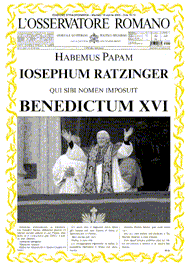Vatican News Paper Osservatore Romano announces the election of Benedictum XVI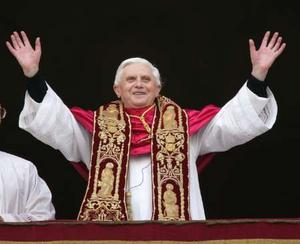Source: REUTERS / Max Rossi
Pope Benedict XVI greets the crowd from the central balcony of St. Peter's Basilica at the Vatican, Tuesday, April 19, 2005

Besides, John Paul II, mystic patriarch, carried a special worship in the Holy Virgin Mary. Now, the multiple associations of the number 13 to the Holy Virgin Mary are established well: On the Supernatural Medal, M of Mary surmount the Holy Cross of Christ, the one to which we associate the figure 13. Moreover, the letter M is also the 13th letter of the alphabet. Besides, 13 words compose the invocation to the Virgin on the Supernatural Medal: Ô Mary, conceived without sin, pray for us who have appeal to you.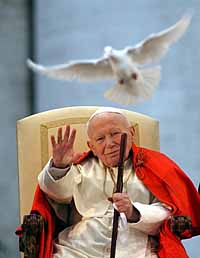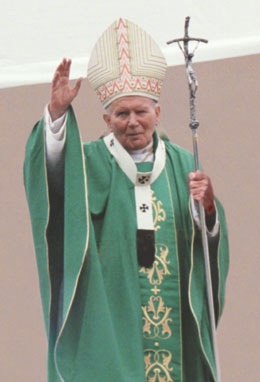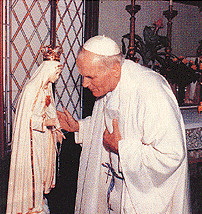Several major and historical acts of Jean-Paul II were deliberately made on the 13th of the month:
(Reference, Vatican, The Holy See - The Holy Father at http://www.vatican.va/holy_father/index.htm )

December 13, 1981
John Paul II invites prayers for the Polish nation, calling the situation a state of emergency.

May 13, 1982
(Exactly 1 year after the attack where he almost lost his life)
Before the statue of Our Lady of Fátima, John Paul II recites the Act of consecration and entrustment of the world to the Immaculate Heart of Mary.

April 13, 1986
John Paul II makes a visit to Rome's main Synagogue .

January 13, 1987
John Paul II receives in audience the President of the Council of the People's Republic of Poland, Wojciech Jaruzelski .

November 13, 1989
Meeting of the diocesan bishops of the Federal Republic of Germany with the Holy Father on the theme: "Handing the faith to the next generation and the pastoral work of catechesis".

April 13, 1991
Reorganization of the Latin-Rite Catholic Church in the Soviet Republic of Belarus, Russia and Kazakistan.

August 13, 1991
Pastoral Visit in Poland, at Czestochowa for the 6th World Youth Day and in Hungary
Apostolic Voyage 52 [52 = 13 x 4]

January 13, 1992
Holy See recognizes Croatia and Slovenia .

February 13, 1992
Papal chirograph for the Indipendent Foundation Popolorum Progressio at the service of the indiginous peoples and the campesinos in Latin America, established on the occasion of the 5th centenary of the evangelization of Latin America.

June 13, 1994
Fifth Plenary Assembly of the College of Cardinals, with John Paul II participating, in preparation for the Jubilee of the Third Millennium.

December 13, 1994
Letter to Children, in the Year of the Family.

November 13, 1996
The Holy Father addresses the opening of the UN World Food Summit organized by FAO (UN Food and Agricultural Organization) in Rome.

May 13, 2000
Cardinal Angelo Sodano announced that the "third part" of the secret of Fatima will be made public. The Message of Fatima will be published in its entirety on 26 [13 x 2] June 2000.

June 13, 2000
John Paul II expresses his satisfaction for the clemency granted to Mehmet Alì Agca [who made an attempt on the Pope's life on May 13, 1981] by the President of Italy during this jubilee year.

December 13, 2001
Famous Meeting of the Pope with Bishops from the Holy Land, on the theme: "Peace in the Holy Land and the Future of Christians"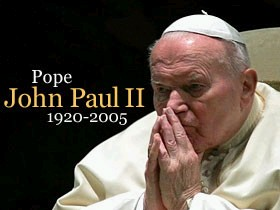## VARIOUS INFORMATIONS

In his title, if we allow to replace Roman II by Latin 2 we obtain:
Pope John Paul 2 = 13 symbols
Pape Jean-Paul 2 = 13 symbols
Giovanni Paolo = 13 symbols
Ioannes Paulus = 13 symbols

The priestly hierarchy of the Roman Catholic Church contains 13th ecclesiastical titles.

The celebration of the Epiphany takes place the 13th day after the nativity of the Lord. Number 13 is called theophanicus for that reason.

The 13th glorious mystery of the Holy Rosary relates directly to the Pentecost.

The number 13 = Numerical value of J.-C. (Jesus Christ), in the logical alphanumeric coding (with C=3 and J=10); A=1, B=2, C=3, D=4,  Y=25, Z=26

In mathematical terms, the number 13 contains the Trinity:
13 = 3/. 3 + 3 = 3: 0,3 + 3 that is 13 = 10 + 3

The number 13 = " Mark of time "; one year = 52 weeks = 13 x 4 weeks.

The alphabet and its language wear the mark of the number 13 because the alphabet contains 26 symbols and 26 = 13 x 2.

### Some others Expressions with sum 13 letters

(See also Friday the 13th (Vendredi 13) at   http://www.vendredi13.co.nr/ )
 Mary MagdaleneThe Holy FatherThe Holy SpiritLe Saint-Esprit (French for The Holy Spirit)The ApocalypseThe ParanormalThe ArmageddonImpossibilityReincarnationMisperceptionThe AppearanceUne apparition(An Appearance in French)Human Implants Pact with devilPact with SatanPacte au diable (Pact with devil in French)Judas IscariotThe Hided PowerLa force cachée (French for The Hided Power)EmbezzlementsBeatificationImperfectionsThe AntichristA «Save Our Souls» (A  S.O.S.)The Microchips The Big BrotherThe Ground ZeroThe Free MasonsKnight TemplarThe Holy BiblesLa Sainte Bible (The Holy Bible in French)Rosslyn ChapelThe invocationMalversations(embezzlements in French)InterdictionsThe MillenniumRoman CatholicThe Kidnapping

## Related URLs:

Friday the 13th (Vendredi 13) at   http://www.vendredi13.co.nr/
666 Myth at   http://www.666myth.co.nr/
666 Mythe at   http://www.666mythe.co.nr/
The Holy See - The Holy Father at http://www.vatican.va/holy_father/index.htm
Pope Benedict XVI, From Wikipedia, the free encyclopedia at http://en.wikipedia.org/wiki/Joseph_Cardinal_Ratzinger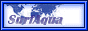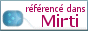The Pheedster Directory     CrossDaily.com